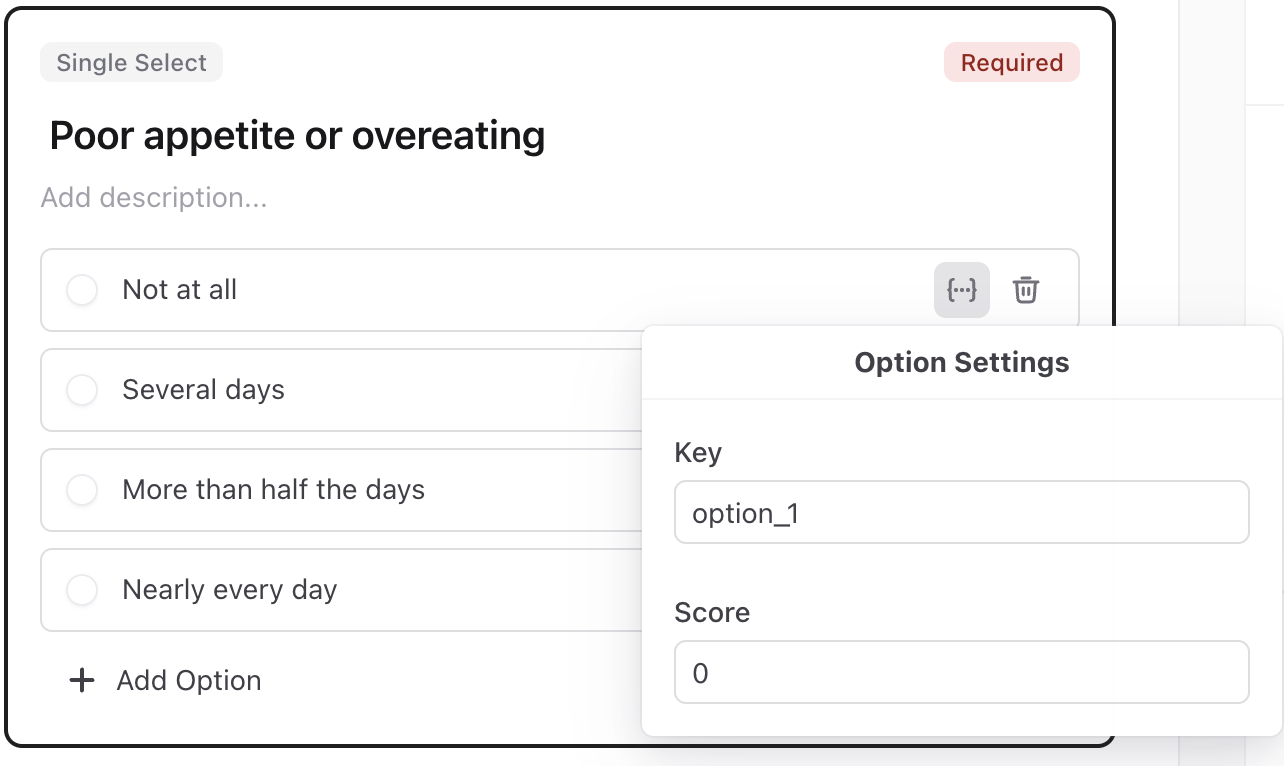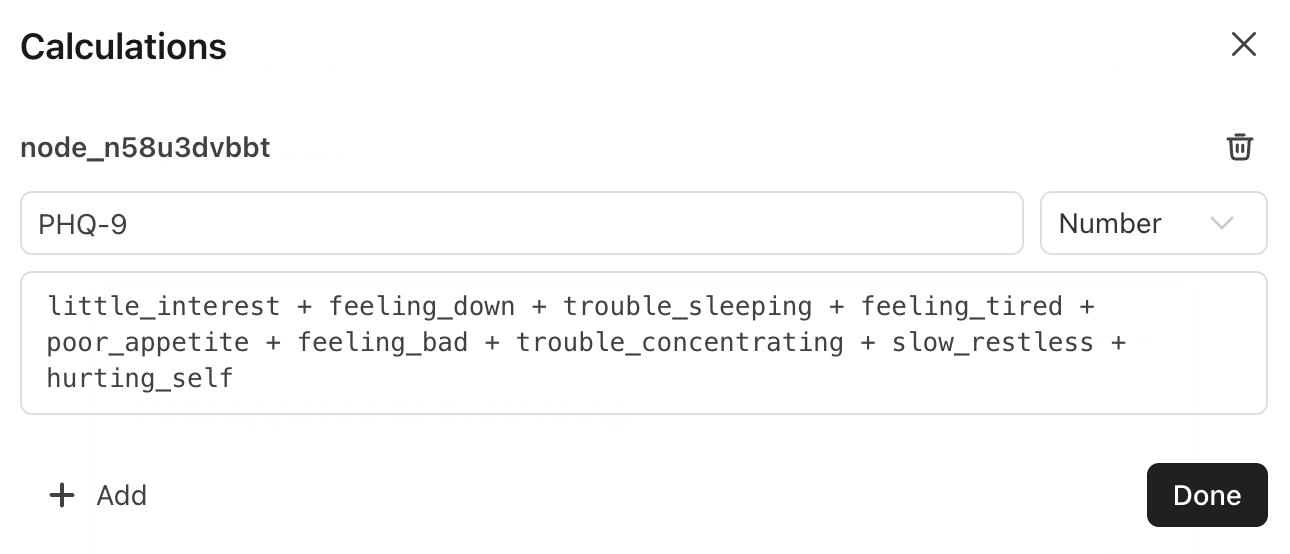# Overview

Calculated fields allow you to generate data within a form based on how a member answers questions within that form. For example, you may wish to add up a form’s responses to calculated a score, as for a PHQ-9 screener. Or you may simply want to calculate the age of a member based on a question about their date of birth.

Form builders can easily create formulas and store the calculated data within the form response. Calculated fields display to your care team within the form response in the Source UI and are available via API for integrations you’ve built.

# Using Calculated Fields

To add a calculated field to a form, first open the form via the Settings (⚙️) > Forms page.

1. Add the questions to your form that you want to use in your calculated field. You can use Single Select and Multi Select question types as part of a calculation by setting a score for each selection
• For Single or Multi Select question, select “Enable option scoring” and next to each option click the score {…} button to add a numeric score1. In the form menu a the top-right of the page, click the calculated field (%) button
3. Set a name for your calculated field, choose its data type, and enter the expression you want to calculate. See below for tips on expressions and for some examples to get started1. You can add multiple calculated fields and delete ones you no longer need
2. Once you’ve added your calculated field, save the form and publish the new version to start capturing the calculated field in form responses

# Writing Expressions

An expression can contain a combination of math symbols + - / * ( ), formulas, and keys for other questions within the form. You can locate the key of a question by clicking on that question in the form builder. Use the examples and formula list below for reference in writing your own expressions.

## Example expressions

• Adding two single-select questions together: `first_question_key + second_question_key`
• Calculating age as an integer: `FLOOR(AGE(date_of_birth_question_key))`
• Return a “yes” string if a question exactly matches a string; otherwise, return “no”: `IF(text_question_key = "blue", "yes" , "no")`

## Formula Reference

Number formulas

• `ABS()` - Returns the absolute value of a number.
• Example: `ABS(number_question_key)`
• `FLOOR()` - Rounds down and returns the largest integer less than or equal to a number.
• Example: `FLOOR(number_question_key)`
• `CEILING()` - Rounds up and returns the smaller integer greater than or equal to a number.
• Example: `CEILING(number_question_key)`
• `ROUND()` - Returns the value of a number rounded to the nearest integer.
• Example: `ROUND(number_question_key)`
• `MAX()` - Returns the largest of the numbers given as parameters.
• Example: `MAX(number_question_key , another_number_question_key)`
• `MIN()` - Returns the smallest of the numbers given as parameters.
• Example: `MIN(number_question_key , another_number_question_key)`

Date formulas

• `AGE()` - Calculates the age of a date, in years.
• Example: `AGE(date_of_birth_question_key)`
• Output type: number
• `DATE()` - Turns and ISO date string into a date object.
• Example: `DATE(string_question_key)`
• Output type: date object
• `DATETIME()` - Turns and ISO date string into a datetime object.
• Example: `DATETIME(string_question_key)`
• Output type: datetime object

Logic formulas

• `IF()` - Evaluates the condition in the first parameter. If true, returns the second parameter. If false, returns the third parameter.
• Example: `IF(string_question_key = "blue" , "string to return if true" , "string to return if false)`
• `IFNULL()` - Returns a fallback value if the provided value is null.
• Example: `IFNULL(string_question_key , "string to return if null")`